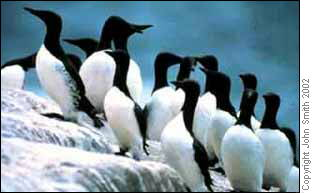Drawing an ImageThis example shows how to draw an image from a file, put a frame round it and then draw some rotated text at the bottom right of the image.
 1Setting Up We start by setting up variables we will use later. These include information on the source file, the path to the output file and some text we are going to draw on the Canvas. [VBScript] ```srcfile = Server.MapPath("imagefile.tiff") dstfile = Server.MapPath("newfile.jpg") text = "Copyright John Smith 2002"```
 2Creating the Canvas Create the Canvas using the standard CreateObject method. We are going to be drawing seven point Arial in gray later on so set these default values now. [VBScript] ```Set canvas = Server.CreateObject("ImageGlue7.Canvas") canvas.TextFont = "Arial" canvas.TextSize = 7 canvas.TextColor = "gray"```
 3Drawing the File Set the width of the canvas to 300 pixels. As no height has been specified for the Canvas DrawFile will have to work one out for us. Because we have specified a width a height will automatically be chosen that maintains the correct aspect ratio of the source image. If the image is smaller than the Canvas, then we will want to draw it in the original size (if we enlarged it, it would end up rather pixelated). To do this we specify the Fit parameter and tell DrawFile that we want the image aligned in the middle (both horizontally and vertically). [VBScript] ```canvas.Width = 300 canvas.DrawFile srcfile, "Fit=True VAlign=middle HAlign=middle"```
 4Drawing a Frame Draw a frame round the edge of the canvas. Because the Rect in the Parameter String defaults to the size of the canvas we just have to ask DrawShape to draw a rect and it will put it in the right place. [VBScript] `canvas.DrawShape "rect", "Operation=draw"`
 5Drawing the Text Because we are going to draw text at the bottom right of the image we first find out how much space it is going to take up and then enlarge the Canvas by this amount. We then draw the text rotated and shifted to the bottom of the Canvas. [VBScript] ```canvas.TextArea text, "", w, h, lh canvas.Width = canvas.Width + h + 2 thetrans = "Rotate=-90 Translate=300," & (canvas.Height - 2) canvas.DrawText text, thetrans```
 6Saving Finally we save the picture as a high quality JPEG image. [VBScript] `canvas.SaveAs dstfile, "Quality=high"`
 7Input and Output Sample input and output images are shown below.imagefile.tiffnewfile.jpg